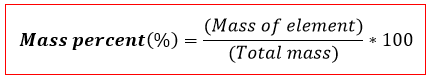# Problem: An unknown compound was determined to be 74.97% carbon, 8.39% hydrogen, and 16.64% oxygen. Calculate the empirical formula.

###### FREE Expert Solution

We’re asked to determine the empirical formula of an unknown compound with 74.97% Carbon (C), 8.39% Hydrogen (H), and 16.64% Oxygen (O). These values are Mass percent of each element.

Remember that Mass or weight percent is the percentage of a given element in a compound:Assuming we have 100 g of the compound, this means we have 74.97 g of C, 8.39 g of H and 16.64 g of O

Their atomic masses are 12 g/mol C, 1 g/mol H and 16 g/mol O.

82% (183 ratings)###### Problem Details

An unknown compound was determined to be 74.97% carbon, 8.39% hydrogen, and 16.64% oxygen. Calculate the empirical formula.